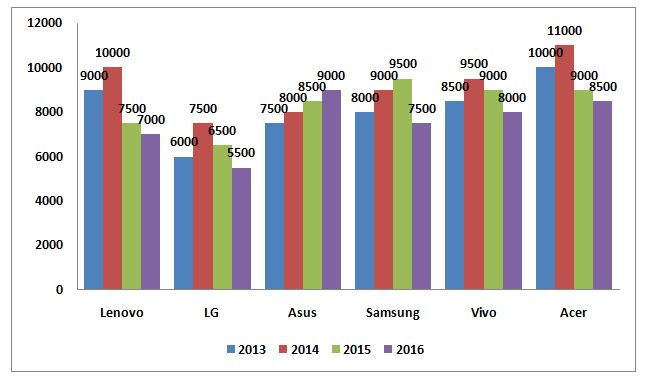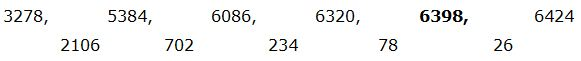# SBI Clerk Prelims 2020 Quantitative Aptitude Questions (Day-10)

Dear Aspirants, Our IBPS Guide team is providing new series of Quantitative Aptitude Questions for SBI Clerk Prelims 2020 so the aspirants can practice it on a daily basis. These questions are framed by our skilled experts after understanding your needs thoroughly. Aspirants can practice these new series questions daily to familiarize with the exact exam pattern and make your preparation effective.

[WpProQuiz 7632]

Ensure Your Ability Before the Exam – Take SBI Clerk 2020 Prelims Free Mock Test

Missing Number series

Directions (Q. 1 – 5): What value should come in place of question mark (?) in the following number series?

1) 7512, 3756, 1252, ? , 62.6

a) 645

b) 728

c) 313

d) 857

e) 934

2) 36, 32, 73, 203, 837, ?

a) 4149

b) 3352

c) 3578

d) 3966

e) 3745

3) 3278, 5384, 6086, 6320, ? , 6424

a) 6382

b) 6406

c) 6354

d) 6398

e) 6412

4) 819, 818, 408, 135, ?

a) 37.25

b) 32.75

c) 48.5

d) 54.25

e) 56.75

5) 1276, 1280, 1296, 1360, ? , 2640

a) 2252

b) 2078

c) 1616

d) 1824

e) 1536

Data Interpretation

Directions (Q. 6 – 10) Study the following information carefully and answer the given questions:

The following bar graph shows the prices (In thousands) of different types of mobiles in different years.6) Find the difference between the price of Lenovo to that of Samsung in all the given years together?

a) 2200

b) 1300

c) 1500

d) 1800

e) None of these

7) Find the ratio between the total price of all the given mobiles in the year 2013 to that in the year 2016?

a) 13 : 7

b) 25 : 14

c) 98 : 91

d) 123 : 109

e) None of these

8) Find the total price of all the given mobiles in the year 2015?

a) 45000

b) 60000

c) 55000

d) 50000

e) None of these

9) The price of LG mobiles in all the given years together is approximately what percentage less than the price of Vivo mobiles in all the given years together?

a) 12 %

b) 27 %

c) 35 %

d) 46 %

e) 54 %

10) The price of all the given mobiles in the year 2014 is approximately what percentage of price of Asus in all the given years together?

a) 142 %

b) 156 %

c) 167 %

d) 130 %

e) 118 %

Directions (1-5):

The pattern is, ÷ 2, ÷ 3, ÷ 4, ÷ 5,….

The pattern is, *1 – 22, *2 + 32, *3 – 42, *4 + 52, *5 – 62,….The difference is, ÷ 3

The pattern is,

(819 – 1)/1 = 818

(818 – 2)/2 = 408

(408 – 3)/3 = 135

(135 – 4)/4 = 32.75

The pattern is, +41, +42, +43, +44,….

Directions (6-10):

The price of Lenovo in all the given years together

= > 9000 + 10000 + 7500 + 7000 = 33500

The price of Samsung in all the given years together

= > 8000 + 9000 + 9500 + 7500 = 32000

Required difference = 33500 – 32000 = 1500

Total price of all the given mobiles in the year 2013

= > 9000 + 6000 + 7500 + 8000 + 8500 + 10000 = 49000

Total price of all the given mobiles in the year 2016

= > 7000 + 5500 + 9000 + 7500 + 8000 + 8500 = 45500

Required ratio = 49000 : 45500 = 98 : 91

The total price of all the given mobiles in the year 2015

= > 7500 + 6500 + 8500 + 9500 + 9000 + 9000

= > 50000

The price of LG mobiles in all the given years together

= > 6000 + 7500 + 6500 + 5500 = 25500

The price of Vivo mobiles in all the given years together

= > 8500 + 9500 + 9000 + 8000 = 35000

Required % = [(35000 – 25500)/35000]*100 = 27 %

The price of all the given mobiles in the year 2014

= > 10000 + 7500 + 8000 + 9000 + 9500 + 11000 = 55000

The price of Asus in all the given years together

= > 7500 + 8000 + 8500 + 9000 = 33000

Required % = (55000/33000)*100 = 166.66 % = 167 %

 Check Here to View SBI Clerk Prelims 2020 Quantitative Aptitude Questions Day 09 Day 08 Day  07 Click Here for SBI Clerk 2020 – Detailed Exam Notification